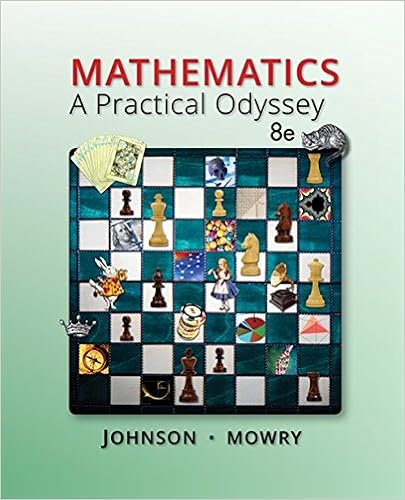# A major department store chain is interested in

• 10
• 88% (41) 36 out of 41 people found this document helpful

This preview shows page 3 - 5 out of 10 pages.

##### We have textbook solutions for you!
The document you are viewing contains questions related to this textbook.The document you are viewing contains questions related to this textbook.
Chapter 4 / Exercise 4
Mathematics: A Practical Odyssey
Johnson/MowryExpert Verified
14) A major department store chain is interested in estimating the mean amount its credit card customersspent on their first visit to the chain's new store in the mall. Fifteen credit card accounts were randomly sampled and analyzed with the following results: = \$50.50 and S = 20. Assuming the distribution of the amount spent on their first visit is approximately normal, what is the shape of the sampling distribution of the sample mean that will be used to create the desired confidence interval for μ?A) approximately normal with a mean of \$50.50B) a standard normal distributionC) a t distribution with 15 degrees of freedomD) a tdistribution with 14 degrees of freedomAnswer:
D15) A major department store chain is interested in estimating the mean amount its credit card customersspent on their first visit to the chain's new store in the mall. Fifteen credit card accounts were randomly sampled and analyzed with the following results: =\$50.50 and S = 20. Construct a 95% confidence interval for the mean amount its credit card customers spent on their first visit to the chain's new store in the mall assuming that the amount spent follows a normal distribution.
3
##### We have textbook solutions for you!
The document you are viewing contains questions related to this textbook.The document you are viewing contains questions related to this textbook.
Chapter 4 / Exercise 4
Mathematics: A Practical Odyssey
Johnson/MowryExpert Verified
D16) Private colleges and universities rely on money contributed by individuals and corporations for their operating expenses. Much of this money is put into a fund called an endowment, and the college spends only the interest earned by the fund. A recent survey of 8 private colleges in the United States revealed the following endowments (in millions of dollars): 60.2, 47.0, 235.1, 490.0, 122.6, 177.5, 95.4, and 220.0. Summary statistics yield = \$180.975 and S = 143.042. Calculate a 95% confidence interval for the mean endowment of all the private colleges in the United States assuming a normal distribution for the endowments.
D17) As an aid to the establishment of personnel requirements, the director of a hospital wishes to estimate the mean number of people who are admitted to the emergency room during a 24-hour period. The director randomly selects 64 different 24-hour periods and determines the number of admissions for each. For this sample, = 19.8 and S= 5. Which of the following assumptions is necessary in order for a confidence interval to be valid?
D
•••